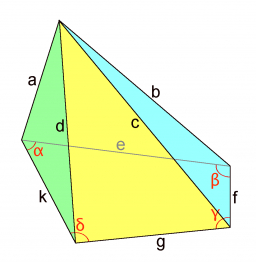# Calculate 73704

A vertical regular 3-sided pyramid is given. The side of the base a = 5cm, and the height is 8 cm. Calculate the volume and area.

V =  86.6025 cm3
S =  73.6868 cm2

### Step-by-step explanation:Did you find an error or inaccuracy? Feel free to write us. Thank you!

Tips for related online calculators
Need help calculating sum, simplifying, or multiplying fractions? Try our fraction calculator.
Tip: Our volume units converter will help you convert volume units.
The Pythagorean theorem is the base for the right triangle calculator.

#### Grade of the word problem:

We encourage you to watch this tutorial video on this math problem: